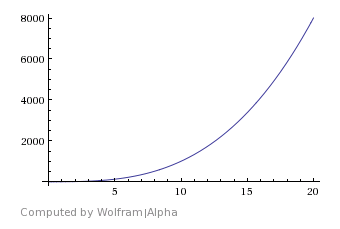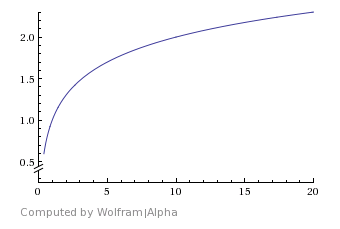July 11, 2013

# Axioms of maintainability

### ax·i·om (noun)

1. A statement or proposition that is regarded as being established, accepted, or self-evidently true.

2. A statement or proposition on which an abstractly defined structure is based.

### Introduction

In Math, axioms are foundational units used to built entire theories classifications upon (e.g. group theory, combinatorics). Without further ado, here are my axioms of maintainability.

### Definitions

`terminal character`, a line feed or end of file `character`.

`line`, an array of `characters` ending with a `terminal character` and not containing 2 or more `terminal characters`.

``````// This is a line, as are the rest in this code fence.

console.log('Hello World!');
``````

`maintenance cost`, metric used to measure how many actions must be taken when an alteration is made to any `character` or set of `characters` in a `line`. This is also known as `cost of maintnence`.

`zero`, the additive identity from group theory.

`non-zero`, an element from a group that is not the additive identity/`zero`.

### Axioms

#### Axiom of emptiness

If a `line` contains no characters except for a `terminal character`, it has `zero` `cost of maintainenance`. The following example is intentionally blank.

``````
``````

#### Axiom of non-emptiness

If a `line` that contains at least one character that is not a `terminal character`, has a `non-zero` `maintenance cost`.

``````// This `line` has a `non-zero` `maintenance cost` as does the next.
console.log('...but I am so innocent ;_;');
``````

Given multiple `lines`, the cumulative `maintenance cost` is the sum of each `line`'s maintenance cost`.

``````// Assume this `line` has a `maintenance cost` of 1
console.log('and this has a `cost` of 5. Then, then the cumulative `cost` is 6');
``````

### Applications

Now that we have a short and sweet set of definitions and axioms, it is time for some applications.

If you didn't notice, the `maintenance cost` is never defined in the theory; the application process should defines how a `cost` is calculated. In most cases, comments and whitespace are "cheap" to maintain:

``````// This line costs `1` unit
// This line costs `2` units since it has leading whitespace and a comment
``````

and a line of code's `cost` depends on its complexity:

``````console.log('In this application, I cost `5` units.');

// but the minified version of jQuery could cost 1000 units.
(function(e,t){var n,r,i=typeof t,o=e.document,a=e.location,s=e.jQuery,u=e.\$,l={},c=[],p="1.9.1",f=c.concat,d=c.push,[...]
``````

The goal should not be to keep the total `maintenance cost` as low as possible but to keep the growth as logarithmic as possible:Logarithmic growth (good):#### Explaining why copy/paste is bad via theory

Let's assume your application looks like:

``````var fs = require('fs'),
http = require('http');

http.createServer(function (req, res) {
// Send a valid status code

// Stream over file to response
}).listen(3000);
``````

We will give that a `maintenance code` of 32 (1 for comments, 5 for line of code). Now, let's say your application begins to get more and more complex:

``````var fs = require('fs'),
http = require('http');

http.createServer(function (req, res) {
if (req.url === '/' && req.method === 'POST') {
res.end();
} else if (req.url === '/') {
} else if (req.url === '/info') {
} else if (req.url === '/info' && req.method === 'PUT') {
} else if (req.url === '/about') {
} else if (req.url === '/secret') {
} else if (req.url === '/more-info.html') {
} else {
res.end();
}
}).listen(3000);
``````

The above has a `maintenance code` of 147 and includes no comments. This is what exponential growth feels like.

Next, imagine what happens if `node` were to deprecate `fs.createReadStream`, almost half of the lines of code would need to be updated. On top of which, each would need to be tested, making everything more painful if you lack automated testing.

#### Explaining why DRY code and abstraction is good via theory

If we DRY up the code via a module like express, normalize all requests to be to `.json`/`.html`, then the maintenance of the above code decreases significantly.

``````var express = require('express'),
app = express();

// Host all local content statically (e.g. 'index.html', 'info.json', 'about.html', 'secret.json')
app.use(express['static'](__dirname + '/public'));

// Start listening for requests
app.listen(3000);
``````

Additionally, if `fs.createReadStream` or any other core `node` methods were to be deprecated, they would be swallowed by `express`, leading to 1 line of updated code for the `package.json`. This is what logaritmic growth feels like.